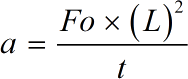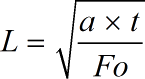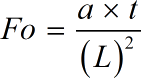Request a Tool

Fourier Number Calculator

This Fourier Number Calculator is used to calculate the characterization of heat conduction.

Output

Thermal Diffusivity
0

Formula• a = Thermal Diffusivity
• Fo = Fourier Number
• L = Characteristic Length
• t = Characteristic Time

Defination / Uses

The phrase "Fourier Number" refers to a dimensionless number that is used to characterise heat conduction. Fourier modulus is another name for the Fourier number. The ratio of the rate of heat conduction to the rate of thermal energy storage is known as the Fourier Number. The symbol for the Fourier Number is 'Fo.' In short, it is a study of unsteady-state mass transfer which is equal to the product of the diffusion coefficient and a characteristic time.

The Fourier Number is a dimensionless number equal to the product of the diffusion coefficient and a characteristic time divided by the square of a characteristic length used in the study of unsteady-state mass transfer.

Output

Characteristic Time
0

Formula• t = Characteristic Time
• a = Thermal Diffusivity
• Fo = Fourier Number
• L = Characteristic Length

Defination / Uses

The phrase "Fourier Number" refers to a dimensionless number that is used to characterise heat conduction. Fourier modulus is another name for the Fourier number. The ratio of the rate of heat conduction to the rate of thermal energy storage is known as the Fourier Number. The symbol for the Fourier Number is 'Fo.' In short, it is a study of unsteady-state mass transfer which is equal to the product of the diffusion coefficient and a characteristic time.

The Fourier Number is a dimensionless number equal to the product of the diffusion coefficient and a characteristic time divided by the square of a characteristic length used in the study of unsteady-state mass transfer.

Output

Characteristic Length
0

Formula• L = Characteristic Length
• t = Characteristic Time
• a = Thermal Diffusivity
• Fo = Fourier Number

Defination / Uses

The phrase "Fourier Number" refers to a dimensionless number that is used to characterise heat conduction. Fourier modulus is another name for the Fourier number. The ratio of the rate of heat conduction to the rate of thermal energy storage is known as the Fourier Number. The symbol for the Fourier Number is 'Fo.' In short, it is a study of unsteady-state mass transfer which is equal to the product of the diffusion coefficient and a characteristic time.

The Fourier Number is a dimensionless number equal to the product of the diffusion coefficient and a characteristic time divided by the square of a characteristic length used in the study of unsteady-state mass transfer.

Fourier Number
0

Formula• Fo = Fourier Number
• L = Characteristic Length
• t = Characteristic Time
• a = Thermal Diffusivity

Defination / Uses

The phrase "Fourier Number" refers to a dimensionless number that is used to characterise heat conduction. Fourier modulus is another name for the Fourier number. The ratio of the rate of heat conduction to the rate of thermal energy storage is known as the Fourier Number. The symbol for the Fourier Number is 'Fo.' In short, it is a study of unsteady-state mass transfer which is equal to the product of the diffusion coefficient and a characteristic time.

The Fourier Number is a dimensionless number equal to the product of the diffusion coefficient and a characteristic time divided by the square of a characteristic length used in the study of unsteady-state mass transfer.

How calculte the fourier number?

There are only two basic steps.

• First select the option from dropdown. What you need to calculate.
• Then add the other values in their corresponding fields.

Thats it! You will get your answer in less than a second. Use upper given formula in case of manual calculation.

In a nutshell, it's a study of unsteady-state mass transfer, where the diffusion coefficient is multiplied by a characteristic time. The Fourier Number is a dimensionless number equal to the product of the diffusion coefficient and a characteristic time divided by the square of a characteristic length used in the study of unsteady-state mass transfer. For quick calculation use our weetools. No sign-up, registration OR captcha is required to use this tool.